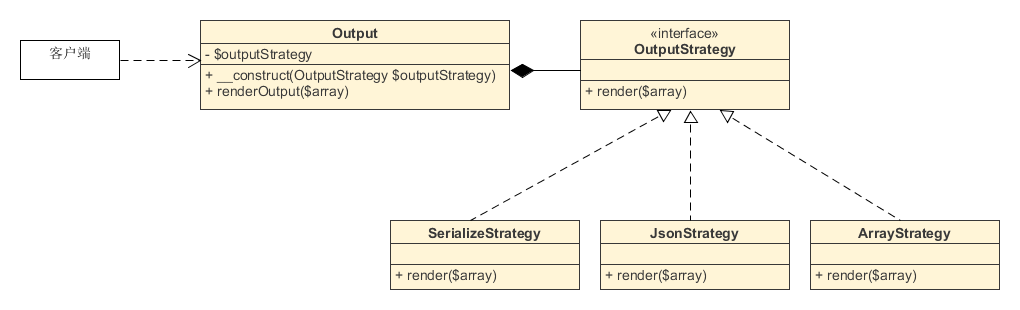# 策略模式

5.7k

## 1 问题

```/**
* 根据给定类型，将数组转换后输出
*/
class Output
{
public function render(\$array, \$type = '')
{
if (\$type === 'serialize') {
return serialize(\$array);
} elseif (\$type === 'json') {
return json_encode(\$array);
} else {
return \$array;
}
}
}```

```/**
* 客户端代码
*/
\$test = ['a', 'b', 'c'];

// 实例化输出类
\$output = new Output();

// 直接返回数组
\$data = \$output->render(\$test, 'array');

// 返回JSON字符串
\$data = \$output->render(\$test, 'json');```

## 2 解决

### 2.1 策略类

```/**
* 策略接口
*/
interface OutputStrategy
{
public function render(\$array);
}

/**
* 策略类1：返回序列化字符串
*/
class SerializeStrategy implements OutputStrategy
{
public function render(\$array)
{
return serialize(\$array);
}
}

/**
* 策略类2：返回JSON编码后的字符串
*/
class JsonStrategy implements OutputStrategy
{
public function render(\$array)
{
return json_encode(\$array);
}
}

/**
* 策略类3：直接返回数组
*/
class ArrayStrategy implements OutputStrategy
{
public function render(\$array)
{
return \$array;
}
}```

（根据FIG-PSR规范，一个类就是一个独立的PHP文件。）

### 2.2 环境类

```/**
* 环境角色类
*/
class Output
{
private \$outputStrategy;

// 传入的参数必须是策略接口的子类或子类的实例
public function __construct(OutputStrategy \$outputStrategy)
{
\$this->outputStrategy = \$outputStrategy;
}

public function renderOutput(\$array)
{
return \$this->outputStrategy->render(\$array);
}
}```

### 2.3 客户端代码

```/**
* 客户端代码
*/
\$test = ['a', 'b', 'c'];

// 需要返回数组
\$output = new Output(new ArrayStrategy());
\$data = \$output->renderOutput(\$test);

// 需要返回JSON
\$output = new Output(new JsonStrategy());
\$data = \$output->renderOutput(\$test);```

## 3 特点M
Maxwell says: 回复